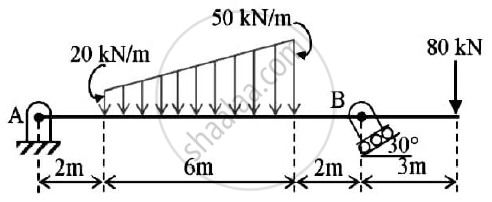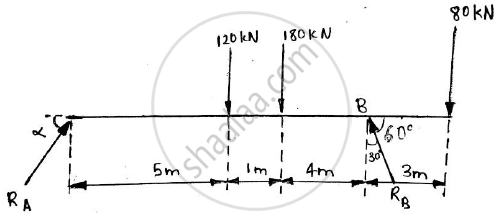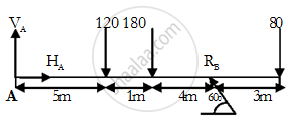# Find the Support Reactions at a and B for the Beam Loaded as Shown in the Given Figure. - Engineering Mechanics

Short Note

Find the support reactions at A and B for the beam loaded as shown in the given figure.#### Solution 1

Given : Various forces on beam
To find : Support reactions at A and B
Solution: Draw PQ ⊥to RS
Effective force of uniform load =20 x 6 = 120 kN
2 + 6/2 = 5 m
This load acts at 5m from A
Effective force of uniformly varying load =1/2 x (80-20) x 6
=180 kN
2+6/3 x 2 =6m
This load acts at 6m from AThe beam is in equilibrium
Applying the conditions of equilibrium
ΣMA = 0
-120 x 5 -180 x 6 +RBcos30 x 10 -80 x 13 =0
10RBcos 30 = 120 x 5+180 x 6 + 80 x13
RB = 314.0785 N
Reaction at B will be at 60o in second quadrant
ΣFx = 0
RAcosα - RBsin30 = 0
RAcosα - 314.0785 x 0.5 = 0
RAcosα = 157.0393 N ……..(1)
ΣFy = 0
RAsinα - 120 - 180 + RBcos30 - 80 = 0
RAsinα = 12 + 180 -314.0785 x0.866 + 80
RAsinα = 108.008N ……..(2)
Squaring and adding (1) and (2)
RA2(sin2α + cos2α) = 36325.3333
RA = 190.5921 N
Dividing (2) by (1)
(R_Asinalpha)/(R_Acosalpha)=(108.008)/(157.0393)
α=tan-1(0.6877)

=34.5173o
Reaction at point A = 190.5921 N at 34.5173o in first quadrant
Reaction at B = 314.0785 N at 60o in second quadrant

#### Solution 2

As Shown in FBD,

Taking two components of RA & taking support reaction at pt. B which inclined at angle 60with horizontal. Also converting the trapezoidal load into equivalent point loads we get FBD

Now , applying conditions of equilibrium

sumF_x = H_A - R_B cos 60 = 0 ------(1)

sumF_Y = V_A - 120 -180-80 +R_B sin 60 = 0 -----(2)

120(5) + 180(6) - RB sin 60(10) + 80(13) = 0

RB = 314.08 KN at θ = 60°

Putting in eqs. (1) and (2), we get

HA = 157.04KN,VA = 108KN

So, R= 190.59 KN  at θ = 34.52°

Support reaction at A is 190.59 KN at θ = 34.52° & at N is RB = 314.08 KN at θ = 60°Concept: Condition of equilibrium for parallel forces
Is there an error in this question or solution?